Our World Statistics Day conversations have been a great reminder of how much statistics can inform our lives. Do you have an example of how statistics has made a difference in your life? Share your story with the Community!
Choose Language Hide Translation Bar
Highlighted

## JMP multiple XY plots in a grid without headings

Hello,

I want to plot multiple XY graphs, representing steel defects over width (X) and length (Y).

I want to arrange them in rows in a grid, by the coilnumber (product number).

I use the following code:

``````Names default to here( 1 );

Fit Group(
Graph Builder(
Size( 100, 300 ),
Show Control Panel( 0 ),
Show Legend( 0 ),
Show Title ( 0 ),
Variables( X( :PercentBr ), Y( :PercentLe ) ),
Elements( Points( X, Y, Legend( 1 ) )),
By( :cl_n )
),
<<{Arrange in Rows( 15 )}
);``````

However I can't get rid of the title.

Ideally I only want to have the coilnumber: eg. '01702557' for the first cell in the picture instead of 'Graph Builder CL_N=01702557'.

This way I can plot more products on one page.

Thanks!

Peter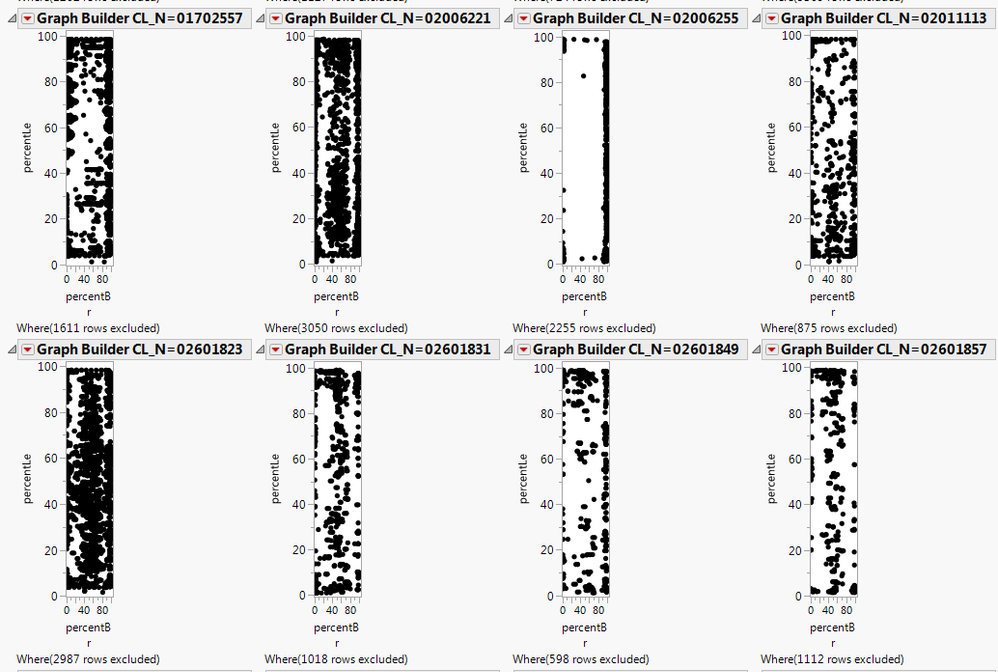2 ACCEPTED SOLUTIONS

Accepted Solutions
Highlighted

## Re: JMP multiple XY plots in a grid without headings

Here is what you asked for.  Not sure it is exactly what you want, but you should be able to see some options on getting it to where you want it.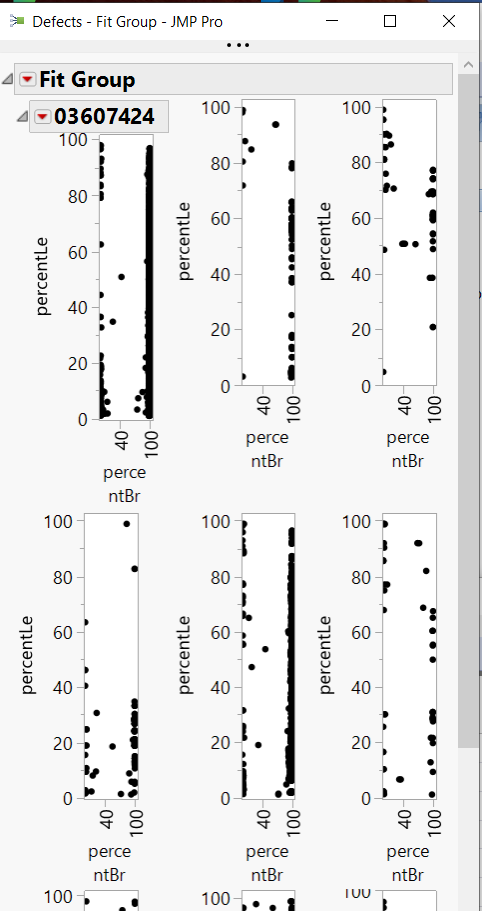``````Names default to here( 1 );

dt=current data table();

summarize(dt,levels=by(:cl_n));

fg=Fit Group(
Graph Builder(
Size( 100, 300 ),
Show Control Panel( 0 ),
Show Legend( 0 ),
Show Title ( 0 ),
Variables( X( :PercentBr ), Y( :PercentLe ) ),
Elements( Points( X, Y, Legend( 1 ) )),
By( :cl_n )
),
<<{Arrange in Rows( 3 )}
);
report(fg)[outlinebox(2)]<<set title(
word(-1,report(fg)[outlinebox(i)]<<get title,"="
)
);

for(i=3,i<=nitems(levels)+1,i++,
report(fg)[outlinebox(i)]<<set title("")
);``````
Jim
Highlighted

## Re: JMP multiple XY plots in a grid without headings

Actually you can drag CL_N to the Wrap role and get this: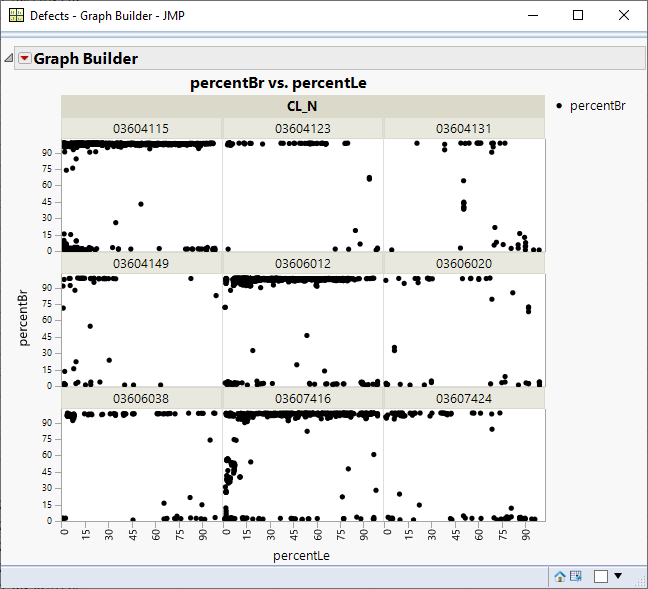Here's the code:

``````Graph Builder(
Size( 1806, 953 ),
Show Control Panel( 0 ),
Variables( X( :percentLe ), Y( :percentBr ), Wrap( :CL_N ) ),
Elements( Points( X, Y, Legend( 13 ) ) )
)``````
6 REPLIES 6
Highlighted

## Re: JMP multiple XY plots in a grid without headings

Can you post some data (real or simulated)?  I have code that does this for adverse events as a function of time.  The R0n and C0n labels are arbitrary row and column labels used to make the plot.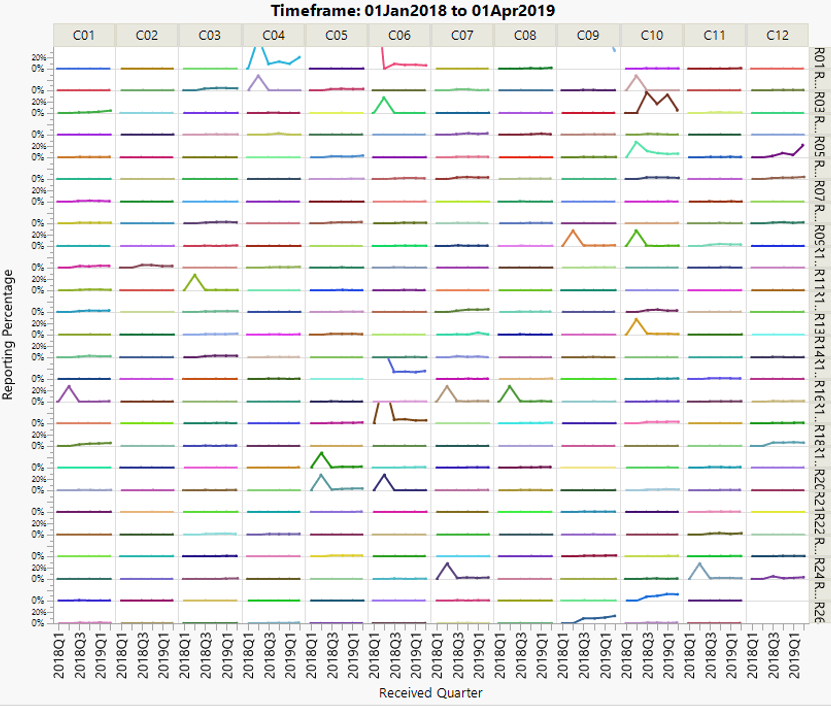Highlighted

## Re: JMP multiple XY plots in a grid without headings

In annex I've added a small sample dataset with 9 coils (products).

For the code I changed the 15 to 3 (this will give a 3x3 matrix).

``````Names default to here( 1 );

Fit Group(
Graph Builder(
Size( 100, 300 ),
Show Control Panel( 0 ),
Show Legend( 0 ),
Show Title ( 0 ),
Variables( X( :PercentBr ), Y( :PercentLe ) ),
Elements( Points( X, Y, Legend( 1 ) )),
By( :cl_n )
),
<<{Arrange in Rows( 3 )}
);``````
Highlighted

## Re: JMP multiple XY plots in a grid without headings

Here is what you asked for.  Not sure it is exactly what you want, but you should be able to see some options on getting it to where you want it.``````Names default to here( 1 );

dt=current data table();

summarize(dt,levels=by(:cl_n));

fg=Fit Group(
Graph Builder(
Size( 100, 300 ),
Show Control Panel( 0 ),
Show Legend( 0 ),
Show Title ( 0 ),
Variables( X( :PercentBr ), Y( :PercentLe ) ),
Elements( Points( X, Y, Legend( 1 ) )),
By( :cl_n )
),
<<{Arrange in Rows( 3 )}
);
report(fg)[outlinebox(2)]<<set title(
word(-1,report(fg)[outlinebox(i)]<<get title,"="
)
);

for(i=3,i<=nitems(levels)+1,i++,
report(fg)[outlinebox(i)]<<set title("")
);``````
Jim
Highlighted

## Re: JMP multiple XY plots in a grid without headings

Actually you can drag CL_N to the Wrap role and get this:Here's the code:

``````Graph Builder(
Size( 1806, 953 ),
Show Control Panel( 0 ),
Variables( X( :percentLe ), Y( :percentBr ), Wrap( :CL_N ) ),
Elements( Points( X, Y, Legend( 13 ) ) )
)``````
Highlighted

## Re: JMP multiple XY plots in a grid without headings

Thanks a lot!

This solution works even better for me.

It is for me a very good way to plot the defects on hundreds of coils in one image: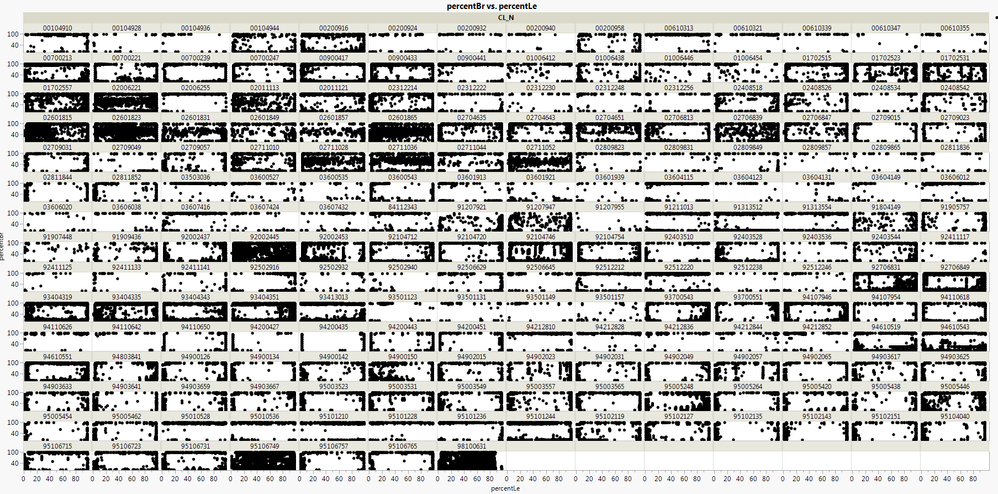Highlighted

## Re: JMP multiple XY plots in a grid without headings

Thanks a lot!
This works fine for me!
Article Labels